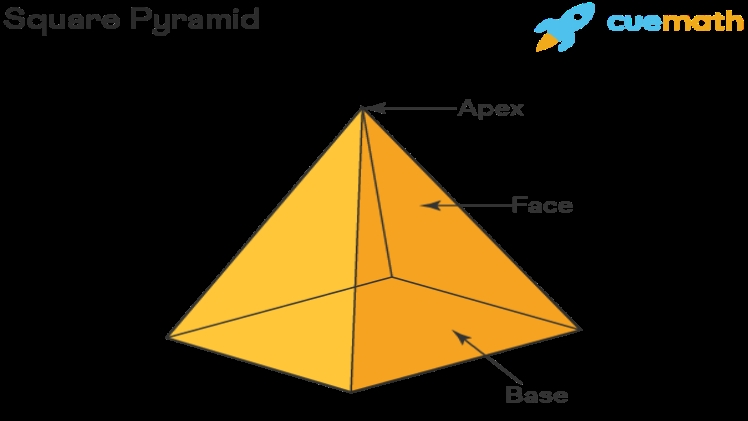# How Many Vertices (corners) Does a Square-based Pyramid Have?A square-based pyramid is a three-dimensional geometric shape that has a square base and four triangular faces. It is a type of pyramid that is based on a square, and it is one of the most common types of pyramids. In this article, we’ll explore how many vertices a square-based pyramid has.

## What is a Square-Based Pyramid?

A square-based pyramid is a three-dimensional geometric shape that has a square base and four triangular faces. It is one of the most common types of pyramids, and it is characterized by its square base and four sides that meet at the apex. The four sides are typically the same size and shape, and each side is a triangle.

## How Many Vertices Does a Square-Based Pyramid Have?

A square-based pyramid has five vertices (corners). The four vertices of the square base and the apex of the pyramid make up the five vertices. The apex is the point at which all four sides of the pyramid meet.

A square-based pyramid is a three-dimensional geometric shape that is characterized by its square base and four triangular faces. It has five vertices, which are the four vertices of the square base and the apex of the pyramid. Knowing how many vertices a square-based pyramid has can help you understand the structure of the shape and how it is formed.